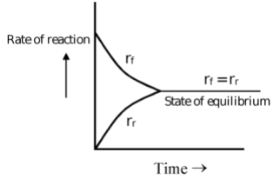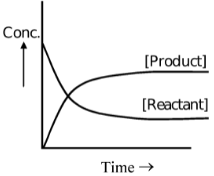# Equilibrium

## Ionic And Chemical Equilibrium of Class 11

If temperature, pressure and composition of a system does not change with time then the system is said to be in thermodynamic equilibrium. It is composed of three different equilibria

(i) Thermal equilibrium: If temperature does not change with time, the system is said to be in thermal equilibrium.

(ii) Mechanical equilibrium: In mechanical equilibrium, the pressure does not change with time.

(iii) Chemical equilibrium: If composition of the system does not change with time, the system is said to be in chemical equilibrium. It is the state in which net reaction of a system is zero.

### Reversible Reactions:

Those reactions in which the reactants transform to give products and products also transform to give reactants are called reversible reactions. Such reactions proceed in both forward and reverse directions but never go to completion in either direction. Such reactions are said to be in chemical equilibrium if the forward and reverse rates are equal. The common examples of such equilibria are

2NO2(g) →  N2O4(g)

H2(g) + I2(g) → 2HI(g)

CH3COOH(l) + C2H5OH(l) →  CH3COOC2H5(l) + H2O(l)

### State of Equilibrium:

In reversible reactions, a stage is reached when the rate of transformation of reactants into products equals the rate of transformation of products into reactants. At this stage, the composition of reactants and products does not change with time. This does not mean that the reaction has ceased, as both reverse and forward reactions are still taking place but with equal pace. Such equilibria are called dynamic equilibria.### Law of Mass Action

According to a law proposed by Guldberg and Waage "at constant temperature, the rate of reaction is directly proportional to active masses of the reactants raised to the power of proper stoichiometric coefficients".

### Active Mass

Active mass of the reactant is directly proportional to its molarity. Active mass of any species is represented by writing the concentration of that species in square brackets.

Active mass of reactant = γ × Molarity

where γ = Activity coefficient

∴ a = γ × Molarity

For very dilute solutions, the value of γ is unity.

∴ a = Molarity